A Novel Wireless Sensor Network Data Aggregation Algorithm Based on Self-organizing Feature Mapping Neutral Network

A Novel Wireless Sensor Network Data Aggregation Algorithm Based on Self-organizing Feature Mapping Neutral Network

Hong Zhou| Kunming Yu

Program in Engineering Science, Chung Hua University, Hsinzhu 30012, Taiwan

Faculty of Computer and Software Engineering, Huaiyin Institute of Technology, Huai'an 223005, China

College of Computer Science and Electrical Engineer, Chung Hua University, Hsinzhu 30012, Taiwan

Corresponding Author Email:
hyitzh@hyit.edu.cn
Page:
119-123
|
DOI:
https://doi.org/10.18280/isi.240118
15 October 2018
|
Accepted:
3 January 2019
|
Published:
20 April 2019
| Citation

OPEN ACCESS

Abstract:

Data aggregation is an efficient method to save energy and prolong the service life of wireless sensor networks (WSNs). In light of this, a data aggregation algorithm was constructed based on self-organizing feature mapping (SOFM) neural network and WSN cluster routing protocol. In this algorithm, each cluster was designed as a three-layer neural network model, which collect a large number of raw data. Then, the feature data were extracted from the raw data, and sent to the sink node. Through a simulation experiment, it is proved that the proposed algorithm, denoted as SOFMDA, outperformed a popular data aggregation method in energy consumption and data accuracy.

Keywords:

wireless sensor networks (WSNs), self-organizing feature mapping (SOFM), neural network, data aggregation, feature extraction

1. Introduction

Recent years has seen the penetration of wireless sensor networks (WSNs) into every corner of our lives. In these networks, every sensor node deployed in the remote environment configures itself without any prior knowledge of network topology, and seeks to monitor the events in its sensing range.

In a typical WSN, multiple sensor nodes are often deployed in the same area to realize full coverage, which brings overlapping sensing ranges. In this case, the data collected by these nodes are very similar due to the high spatial correlation. The storage, calculation and transmission of these redundant data waste a large amount of resources in the network, including storage space and transmission energy, shorten the service life of the entire network, and incur unnecessary computing costs to backend data processing. As a result, the application of WSNs is constrained by limited nodal energy, storage space and computing power. This calls for an effective way to reduce energy consumption and prolong the service life of the networks.

In view of the above, this paper puts forward a WSN data aggregation algorithm based on self-organizing feature mapping (SOFM) neural network, and denotes the proposed algorithm as the SOFMDA. Considering the location-based clustering routing protocol in the WSN, the intra-cluster nodes were taken as the input layer neurons of the SOFM neural network, while the cluster head nodes, relying on the SOFM neural network, classify the data and extract data features, and then send the feature data to the sink node, thereby reducing the transmission load and energy consumption.

2. Literature Review

2.1 SOFM neural network

Artificial neural networks (ANNs) are mathematical models for information processing of structures similar to the synaptic connections in the human brain . The models usually consist of numerous nodes (neurons) and weighted connections between the nodes. Mimicking the brain activities, the ANNs are capable of tackling problems with limited information under multiple constraints, thanks to the powerful abilities of nonlinear approximation, distributed storage, pattern classification and identification.

Despite the popularity of supervised learning rules in ANNs, the SOFM neural network adopts unsupervised learning rules for network training. In this neural network, the internal laws are self-defined through repeated observation, analysis and comparison of objective events, rather than through prediction of correct models (i.e. training inputs/outputs), and the objects are classified by feature similarity. In other words, the training of SOFM neural network only requires a set of inputs because the network can automatically extract the intrinsic features of the inputs to complete the classification task. The structure of the SOFM neural network is presented in Figure 1 below.

fig.1.png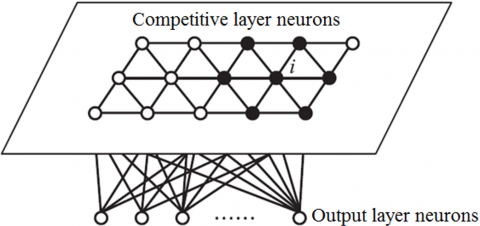Figure 1. Structure of SOFM neural network

The SOFM neural network uses the Kohonen’s learning rule: each winning neuron, i.e. neuron whose weight vector lies closest to the input vector, and all the neurons in its neighborhood are subjected to weight update. Taking Figure 1 for instance, the weights of winning neuron (nodei) and the nodes in its neighborhood (the other solid nodes in the figure) should be updated. This learning rule is similar to the neurons operation in the human brain. The weight update of the Kohonen’s learning rule can be expressed as:

$iw(q)=iw(q-1)+α(p(q)-iw(q-1))$(1)

where q is the number of training rounds; w is marked as weight; α is the learning rate correlated to the learning effect and computing load. If α is too large, the learning results may be poor due to insufficient training; if α is too small, the network may not be able to achieve the learning goals in the long run.

After multiple training rounds, the weights near the winning neuron will approximate the input mode. Therefore, the SOFM is a neural network capable of retaining the input mode and input data topology.

2.2 Data aggregation methods

Heidari et al.  put forward a WSN data aggregation algorithm to overcome the defects of the low energy adaptive clustering hierarchy (LEACH) algorithm in cluster head selection, data aggregation and routing between cluster head and the base station, and verified the improvement effect through simulation experiments. In the WSN data aggregation algorithm, energy control conditions are added to the cluster head selection, and the routing between cluster head and the base station is replaced with a multi-hop reverse multicast tree suitable for data aggregation.

Based on spatial correlations, Wang et al.  developed a data aggregation algorithm that classifies and fuses sensor data according to the spatial location and data features, and proved that the algorithm outperforms the reliability assessment algorithm (RAA) in energy consumption, data acquisition and aggregation effect.

Akkaya  presented a data-centric aggregation technique, i.e. a fuzzy clustering (VF) algorithm, based on Voronoi diagram, and confirmed through simulation experiments that the algorithm can effectively improves the network performance. In this algorithm, the data are aggregated at the cluster head, in light of the residual nodal energy, the service quality and the distance between the cluster head and the neighbor node.

2.3 Application of neural networks in WSNs

Much research has been done on the application of neural networks in WSNs. For instance, Sharaf et al.  uses multi-layer perceptron (MLP) neural networks to identify abnormal events based on the WSN signal changes. Attea  created an adaptive routing algorithm for the WSN based on neural network, and verified through simulation experiments that the algorithm is 0.8 times more efficient than the efficient multi-hop hierarchical routing (EMHR) algorithm; in this algorithm, the cluster head is selected through neural network adaptive learning at the base station and the next hop in the shortest path is determined according to the optimal weight function.

Barbancho et al.  applied SOFM neural network to the WSN for the first time, and proposed a wireless sensor routing protocol based on the SOFM, which fails to consider data aggregation. Sung et al.  completed data aggregation using a three-layer perceptron neural network, which is developed from backpropagation (BP) neural network and WSN clustering routing protocol, and discovered that the neural network can outshine the LEACH algorithm if there is a training output set . Inspired by Reference , Zhang et al.  introduced the processing of data features to the LEACH algorithm and found that the improved algorithm cannot work without training output set.

None of the existing methods can effectively achieve good results through neural network training. Considering the above, this paper introduces the SOFM neural network to the WSN for data aggregation, taking the WSN nodes as neurons in the neural network and the collected data as the neuron inputs. The incremental learning of the neural network was performed in the cluster head before data classification. Such a neural network with unsupervised learning rules was adopted because it is impossible to know the correct classification of training data in advance.

3. Sofmda

The SOFMDA algorithm was designed for real-time monitoring applications like large-scale greenhouse monitoring networks and air quality monitoring networks. In these application scenarios, sensor nodes work continuously, collecting a huge amount of monitoring data. All these data are sent to the sink node for aggregation. However, the data received by the sink node are heavily redundant because of the high similarity between the short-term data collected by each node and the data collected by neighboring nodes in the same period. This paper attempts to eliminate the data redundancy resulted from all kinds of factors in addition to the data similarity. Some possible factors include the light intensity of sensor nodes (greenhouse monitoring) and wind speed (forest fire monitoring). For simplicity, it is assumed that N sensor nodes are randomly distributed in a rectangular sensing area. The symbols and their meanings of the SOFMDA are listed in Table 1 below.

Table 1. The symbols and their meanings of the SOFMDA

 Symbol Meaning N Number of nodes Sink The unique sink node C The set of all clusters Ci The i-th cluster Ci-Head Cluster head of the i-th cluster

3.1 SOFMDA model

The SOFMDA only works under clustering routing protocol. In view of the fairness principle for the selection of cluster head, the routing protocol was partially generalized and the problem was discussed at time T in network topology.

Let C be the set of all clusters at time T in the network topology, M be the number of clusters in the network at that time (M=||C||), Ci be the i-th cluster (CiÍC, i=0,1,…,M-1) (||Ci||=ni), Ci-head be the number of the cluster head nodes of Ci, and e1,e2,…,ei be the causes of data redundancy in the network.

In the SOFMDA, the neural network classification and data aggregation are performed each cluster head. There are Qi=ni input vectors (including data collected by Ci) for the neural network of cluster head Ci-head. Due to the use of SOFM neural network, the Ci-head does not require the correct output for the training set.

Assuming that each input vector has r elements, the input matrix of Ci-head can be expressed as a matrix 2-D with r rows and Qi columns as follows:

$\text{P}=\left[ \begin{matrix} {{e}_{11}} & {{e}_{12}} & \cdots & {{e}_{1{{Q}_{i}}}} \\ {{e}_{21}} & {{e}_{22}} & \cdots & {{e}_{2{{Q}_{i}}}} \\ \vdots & \cdots & \cdots & \vdots \\ {{e}_{r1}} & {{e}_{r2}} & \cdots & {{e}_{r{{Q}_{i}}}} \\ \end{matrix} \right]$ (2)

To classify the vectors, the network weights are trained in cluster Ci through the Qi input column vectors.

fig.2.png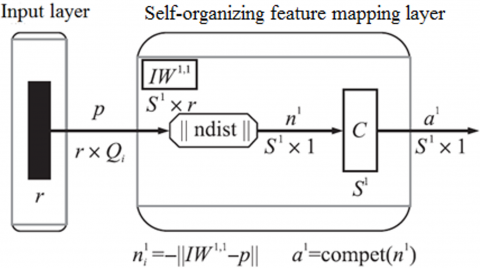Figure 2. Neural network structure in Ci-head

The structure of the SOFM neural network in Ci-head is shown in Figure 2, where IW11 are the weights and threshold matrices of neural networks, ||ndist|| is the error between the input vector p and IW11, S1 is the final number of sets, and a1 is the final classification result.

Through the SOFM neural network in Ci-head, the collected data were classified into S1 sets. The data in each set have a high degree of correlation and can be aggregated by the method specified below.

The SOFMDA data aggregation (DA) process is illustrated in Figure 3, where ci1,ci2,…,cik are the member nodes of cluster Ci (k=Qi). The data classification at the cluster head (Figure 2) is the core part of the SOFMA. The original data were classified into s sets through the SOFM, which are denoted as a1,a2,…,as. With high correlations, the data in the same set are suitable for feature extraction. Taking the s sets as input data for aggregation, the feature values were extracted from the input data and sent to the sink node. The data sent by the cluster node to the cluster head at time t can be expressed as a r+1 tuple:

$P^t_j=(e_1,e_2,e_3,…,e_r,data_j)_t$ (3)

where the first r elements are the redundancy causes used to classify the data at the cluster head; the last element dataj is the real data collected by the sensor. The real data should be aggregated at the cluster head to obtain the final feature data.

fig.3.png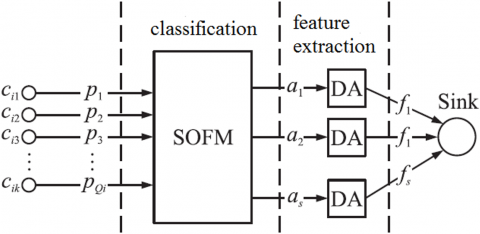Figure 3. SOFM data aggregation model

In the SOFM neural network, the internal weights and threshold matrices should be adjusted continuously with the growth in input samples, such as to accurately classify the input data. Since its initial results may not be sufficiently accurate, the SOFM neural network should receive self-training for a period of time. Normally, the network needs to be trained by a sample for about 200 rounds.

3.2 Feature extraction in SOFMDA

Feature extraction refers to the extraction of the feature value that can represent a type of data. The feature value is either the arithmetic mean (case 1) or the minimum second-order center distance (case 2) of all data. In SOFMDA, the feature value of case 1 can be expressed as:

$f_i=DA(a_i )=\frac{∑_{j=1}^{m_i}data_{i,j}}{n_i}, n_i=||a_i||$ (4)

where ||ai|| is the number of tuples in the set ai; datai,j is the data of the j-th tuple in the set ai; fi is the final data sent by the cluster head ci to the sink node.

Despite its clear meanings, the feature value in case 1 cannot reflect the overall data features accurately if the data vary in a large range, and may be incorrect if there are large singular values. In other words, the feature value in case 1 is robust only if the data are stable and change only in a small range.

Let d be the final data sent to the sink node. Then, the feature value of case 2 can be obtained as:

$f_i=DA(a_i )=∑_{j=1}^{n_i}(data_{i,j}-d)^2 , n_i=||a_i||$ (5)

This feature value can accurately reflect the effect of singular values on the overall data features. However, the cluster head needs high computing power and the operation time is too long in this case. In general, the feature value in case 2 is not suitable for energy-intensive WSNs, unless in special applications.

In addition to the above two cases, the feature value for the SOFMDA can also be obtained by feature extraction methods like flow distribution weighted aggregation algorithm . By this algorithm, the routes are selected based on the network traffic distribution, for the purpose of energy reduction.

Through the above analysis, the SOFM neural network and the traditional data aggregation algorithm of the WSN were combined into a novel WSN data aggregation algorithm.

4. Simulation Experiment

The proposed SOFMDA was applied to an environmental monitoring system through a simulation experiment on Matlab. A total of 100 sensor nodes were arranged in a 100m×100m sensing area to collect the real-time data on temperature and humidity, and send these data to the sink node. Then, these data were classified by the SOFMDA into 4 or 6 sets. The classic LEACH protocol was adopted for this experiment. The experimental parameters are listed in Table 2 below.

Table 2. SOFMDA parameters

 Parameter Value Network range 100m´100m Number of nodes 100 Sink node coordinates (50,50) Initial energy 0.6J Routing protocol LEACH Packet length 4,000 bit Cluster message length 100 bit Header length 100 bit d0 86.7m Eelec 50nJ/bit εfs 10pJ/(bit×m2) εmp 0.0012pJ/(bit×m2) EDA 5nJ/(bit×signal) Redundancy causes Time, position coordinates SOFM training rounds 12 Maximum number of iterations 1600 Simulation time 200s

In the experimental environment, time and space are the leading causes of data redundancy. The SOFMDA measures the energy consumption of data transmission, reception and aggregation by the energy consumption model . The free-space model was adopted if the data transmission distance is below the threshold d0, while the multipath attenuation model was employed if otherwise. The nodal energy consumption of sending and receiving 1 bit data can be calculated as:

${{E}_{Tx}}(l,d)=\left\{ \begin{matrix} l\times {{E}_{elec}}+l\times {{\varepsilon }_{fs}}\times {{d}^{2}} & d<{{d}_{0}} \\ l\times {{E}_{elec}}+l\times {{\varepsilon }_{mp}}\times {{d}^{4}} & d\ge {{d}_{0}} \\\end{matrix} \right.$ (6)

$E_{Rx} (l)=l×E_{elec}$ (7)

where d is the transmission distance; Eelec is the energy consumption of the wireless transceiver circuit for sending or receiving the data per unit of length; εfs and εmp are the energy consumption parameters of the amplifier for the free-space model and the multipath attenuation model, respectively.

The collected data were normalized into the [0, 1] interval before classification, such that the final result would not be affected by possible individual singular values. The network distribution of the 100 sensor nodes is presented in Figure 4. It can be seen that the nodes were scattered randomly in space; the closer the nodes, the more redundant data acquired by them; there are 13 cluster heads in the network.

fig.4.png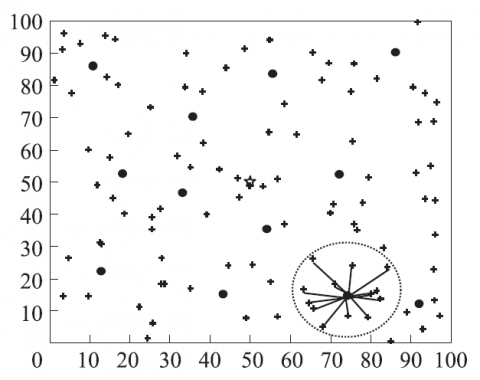Figure 4. Network distribution of 100 nodes

As shown in Figure 5, the data of each cluster head at a certain time were classified by the SOFM neural network into 6 sets (3, 3, 0, 2, 2 and 3) or 4 sets (1, 5, 3 and 4). The number of classified sets bears on the accuracy and integration of data.

fig.5.png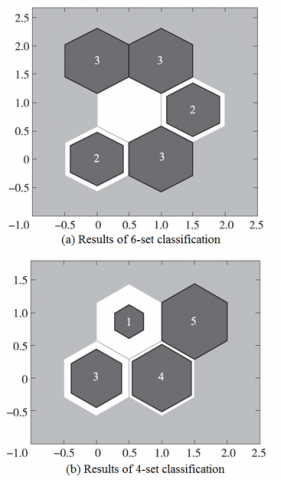Figure 5. Data classification results

Figure 6 shows the network error ratios of 4-set and 6-set classifications. It can be seen that the 6-set classification had a lower error ratio than the 4-set classification. The number of classified sets is positively correlated with the data correlation of each set and the similarity between the feature value and the original data. In addition, the data error was high when the network was just established. This is because the SOFM neural network had a large error at the beginning of learning. With the continued supply of training data, the error of the network gradually reduced and stabilized through incremental learning.

fig.6.png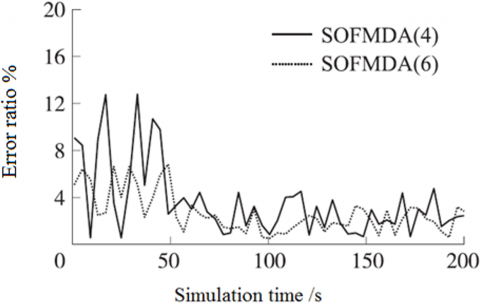Figure 6. Network error ratios of 4-set and 6-set classifications

Figure 7 compares the LEACH and the SOFMDA in energy consumption. It is clear that the SOFMDA consumed less energy than the LEACH. Moreover, the energy consumption of 4-set classification was lower than that of 6-set classification. This is because 6-set classification has a greater degree of data aggregation than 4-set classification, leading to a smaller feature value and lower energy consumption in communication.

fig.7.png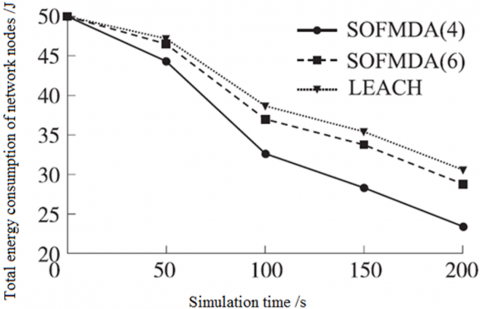Figure 7. Energy consumptions of LEACH and SOFMDA

In light of the above analysis, the number of classified sets in the SOFMDA should be selected to strike a balance between accuracy and energy consumption. Since the CPU needs much more energy than wireless transmission, it makes sense to ensure the computing power at the sake of transmission volume.

5. Conclusions

This paper proposes a WSN data aggregation algorithm based on SOFM neural network, and denotes it as the SOFMDA. Considering the location-based clustering routing protocol in the WSN, the intra-cluster nodes were taken as the input layer neurons of the SOFM neural network, while the cluster head nodes, relying on the SOFM neural network, classify the data and extract data features, and then send the feature data to the sink node, thereby reducing the transmission load and energy consumption. Through a simulation experiment, it is proved that the proposed SOFMDA can effectively reduce the transmission volume through the classification of original data and the extraction of similar data features. The research findings shed new light on energy reduction, channel pressure relief, channel utilization improvement and service life extension of WSNs.

References

 Heidemann J, Silva F, Intanagonwiwat C, Govindan R, Estrin D, Ganesan D. (2001). Building efficient wireless sensor networks with low-level naming. Acm Sigops Operating Systems Review 35(5): 146-159. https://doi.org/10.1145/502034.502049

 Heidari E, Movaghar A, Mahramian M. (2010). The usage of genetic algorithm in clustering and routing in wireless sensor networks. Advances in Intelligent & Soft Computing 67: 95-103. https://doi.org/10.1007/978-3-642-10687-3_9

 Wang YH, Lin YW, Lin YY, Chang HM. (2013). A grid-based clustering routing protocol for wireless sensor networks. Advances in Intelligent Systems and Applications 1: 491-499. https://doi.org/10.1007/978-3-642-35452-6_50

 Akkaya K, Demirbas M, Aygun RS. (2010). The impact of data aggregation on the performance of wireless sensor networks. Wireless Communications & Mobile Computing 8(2): 171-193. https://doi.org/10.1002/wcm.454

 Sharaf MA, Beaver J, Labrinidis A, Chrysanthis PK. (2004). Balancing energy efficiency and quality of aggregate data in sensor networks. Vldb Journal 13(4): 384-403. https://doi.org/10.1007/s00778-004-0138-0

 Attea BA, Khalil EA. (2012). A new evolutionary based routing protocol for clustered heterogeneous wireless sensor networks. Applied Soft Computing Journal 12(7): 1950-1957. https://doi.org/10.1016/j.asoc.2011.04.007

 Barbancho J, León C, Molina FJ, Barbancho A. (2007). Using artificial intelligence in routing schemes for wireless networks. Computer Communications 30(14): 2802-2811. https://doi.org/10.1016/j.comcom.2007.05.023

 Sung WT. (2010). Multi-sensors data fusion system for wireless sensors networks of factory monitoring via BPN technology. Expert Systems with Applications 37(3): 2124-2131. https://doi.org/10.1016/j.eswa.2009.07.062

 Guhaneogi SA, Bhaskar A, Chakrabarti P. (2014). Energy efficient hierarchy-based clustering routing protocol for wireless sensor networks. International Journal of Computer Applications 95(13): 1-8. https://doi.org/10.5120/16651-6627

 Zhang SK, Cui Z.M, Gong SR, Sun Y, Fang W. (2009). A data fusion algorithm based on bayes sequential estimation for wireless sensor network. Journal of Electronics & Information Technology 31(3): 716-721. https://doi.org/10.1360/972009-1549

 Cai ZY, Liu CM, Liu Y, Ye QD. (2013). Application of high performance data fusion algorithm in wireless sensor network. Journal of Qingdao University of Science and Technology (Natural Science Edition) 34(3): 309-314. https://doi.org/10.3969/j.issn.1672-6987.2013.03.019

 Heinzelman WB, Chandrakasan AP, Balakrishnan H. (2002). An application-specific protocol architecture for wireless microsensor networks. IEEE Trans Wireless Commun 1(4): 660-670. https://doi.org/10.1109/TWC.2002.804190